# RS Aggarwal Class 7 Solutions Chapter 20 Mensuration Ex 20A

## RS Aggarwal Class 7 Solutions Chapter 20 Mensuration Ex 20A

These Solutions are part of RS Aggarwal Solutions Class 7. Here we have given RS Aggarwal Solutions Class 7 Chapter 20 Mensuration Ex 20A.

Other Exercises

Question 1.
Solution:
Length of rectangle (l) = 24.5 m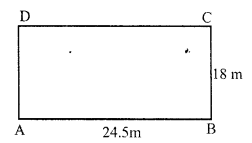Area = l x b = 24.5 x 18 m² = 441 m²
(ii) Length of rectangle (l) = 12.5 m
Breadth (b) = 8 cm = 0.80 m
Area = l x b = 12.5 x 0.80 m² = 10 m²

Question 2.
Solution:
Length of rectangular plot (l) = 48m
and its diagonal = 50m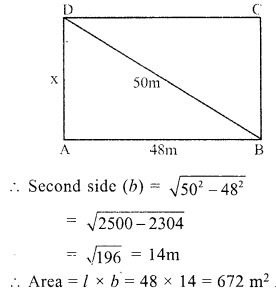Question 3.
Solution:
Ratio in the sides of a rectangle = 4 : 3
Area = 1728 cm²
Let length = 4x,
Area = l x b
1728 = 4x x 3x
⇒ 12x² = 1728
⇒ x² = 144 = (12)²
⇒ x = 12
Length = 4x = 4 x 12 = 48 m
and breadth = 3m = 3 x 12 = 36m
Now perimeter = 2 (l + b) = 2 (48 + 36) m = 2 x 84 = 168 m
Rate of fencing = Rs. 30 per metre
Total cost= 168 x 30 = Rs. 5040

Question 4.
Solution:
Area of rectangular field = 3584 m²
Length = 64 m
Area = 3584
Breadth = $$\frac { Area }{ Length }$$ = $$\frac { 3584 }{ 64 }$$ = 56 m
Now perimeter = 2 (l + b) = 2 (64 + 56) m = 2 x 120 = 240 m
Distance covered in 5 rounds = 240 x 5 = 1200 m
Speed = 6 km/h
Time take = $$\frac { 1200 }{ 1000 }$$ x $$\frac { 60 }{ 6 }$$ = 12 minutes (1 hour = 60 minutes)

Question 5.
Solution:
Length of verandah (l) = 40m
Area = l x b = 40 x 15 = 600m²
Length of one stone = 6dm = $$\frac { 6 }{ 10 }$$ m
and breadth = 5 dm = $$\frac { 5 }{ 10 }$$ m
Area of one stone = $$\frac { 6 }{ 10 }$$ x $$\frac { 5 }{ 10 }$$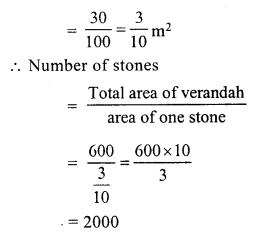Question 6.
Solution:
Length of a room = 13 m
Area of floor = l x b = 13 x 9 m² = 117 m²
or area of carpet = 117 m²
Width = 75 cm = $$\frac { 75 }{ 100 }$$ = $$\frac { 3 }{ 4 }$$ m
Length of carpet = Area ÷ Width
= 117 ÷ $$\frac { 3 }{ 4 }$$
= 117 x $$\frac { 4 }{ 3 }$$ m
= 39 x 4 = 156 m
Rate = Rs. 105 per m
Total cost = Rs. 156 x 105 = Rs. 16380

Question 7.
Solution:
Cost of carpeting a room = Rs. 19200
Rate = Rs. 80 per m
Length of carpet = $$\frac { 19200 }{ 80 }$$ m = 240 m
Width of carpet = 75 cm = $$\frac { 75 }{ 100 }$$ = $$\frac { 3 }{ 4 }$$ m
Area of carpet = 240 x $$\frac { 3 }{ 4 }$$ = 180 m²
Length of a room = 15 m
Width = $$\frac { Area }{ Length }$$ = $$\frac { 180 }{ 15 }$$ = 12m

Question 8.
Solution:
Ratio in length and breadth of a rectangular piece of land = 5 : 3
Cost of fencing = Rs. 9600
and rate = Rs. 24 per m
Perimeter = $$\frac { 9600 }{ 24 }$$ = 400 m
Let length = 5x
Perimeter = 2 (l + b)
⇒ 400 = 2 (5x + 3x)
⇒ 400 = 2 x 8x = 16x
⇒ 16x = 400
⇒ x = 25
Length of the land = 5x = 5 x 25 = 125 m
and width = 3x = 3 x 25 = 75 m

Question 9.
Solution:
Length of hall (l) = 10m.
and height (h) = 5m
Longest pole which can be placed in it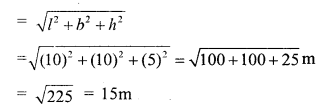Question 10.
Solution:
Side of square (a) = 8.5m
Area = a² = (8.5)² = 8.5 x 8.5 m² = 72.25 m²

Question 11.
Solution:
(i) Length of diagonal of square = 72 cm.
Let length of side = a
Then diagonal = √2 a.
√2 a = 72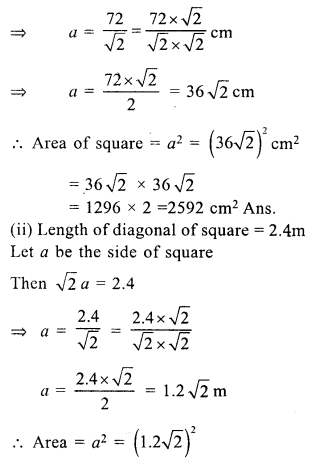= 1.2 x √2 x 1.2 x √2 m²
= 1.44 x 2 = 2.88 m²

Question 12.
Solution:
Area of a square = 16200 m²
Side = √16200 m = √(8100 x 2) m = 90√2 m
Length of diagonal = √2 (side) = √2 x 90√2 = 180 m

Question 13.
Solution:
Area of square = $$\frac { 1 }{ 2 }$$ hectare
= $$\frac { 1 }{ 2 }$$ x 10000 m² = 5000 m²
side (a) = √Area = √5000 m = √(2500 x 2) = 50√2 m
Length of diagonal = √2 (side) = √2 x 50√2 = 100 m

Question 14.
Solution:
Area of sphere plot = 6084 m²
Side (a) = √Area = √6084 m = 78m
Perimeter = 4a = 4 x 78 = 312 m
Length of boundary four times = 312 x 4 = 1248 m

Question 15.
Solution:
Side of a square wire = 10 cm
Perimeter = 4a = 4 x 10 cm = 40 cm
or perimeter of rectangle = 40 cm
Length of rectangle = 12 cm
Breadth = $$\frac { 40 }{ 2 }$$ – 12 = 20 – 12 = 8 cm
Now area of square = a² = (10)² = 100 cm²
and area of rectangle = l x b = 12 x 8 = 96 cm²
Difference in areas = 100 – 96 = 4 cm²
Square has 4 cm² more area

Question 16.
Solution:
Length of go down (l) = 50 m
and height (h) = 10 m
Area of 4 walls = 2 (l + b) x h
= 2 (50 + 40) x 10 m
= 2 x 90 x 10 = 1800 m²
and area of ceiling = l x b = 50 x 40 = 2000 m²
Total area of walls and ceiling = 1800 + 2000 = 3800 m²
Rate of whitewashing = Rs. 20 per m²
Total cost = Rs. 20 x 3800 = Rs. 76000

Question 17.
Solution:
Area of 4 walls of a room= 168m²
Breadth of the room (b) = 10m
and height (h) = 4m.
Let l be the length of room
2 (l + b) h = 168
⇒ 2 (l + 10) x 4 = 168
⇒ l + 10 = $$\frac { 168 }{ 2 x 4 }$$ = 21
⇒ l = 21 – 10 = 11m
Length of the room = 11 m

Question 18.
Solution:
Area of 4 walls of a room = 77 m²
Length of room (l) = 7.5 m
and breadth (b) = 3.5 m
Let h be the height,
then area of four walls = 2 (l + b) h
= 2 (7.5 + 3.5) h = 77
⇒ 2 x 11 x h = 77
⇒ h = $$\frac { 77 }{ 2 x 11 }$$
Height of room = 3.5 m

Question 19.
Solution:
Area of 4 walls = 120 m²
Height (h) = 4m.
and length (l) = 2x
Area of 4 walls = 2 (l + h) x h = 2(2x + x) x 4 = 8 x 3x = 24x
24x = 120
x = $$\frac { 120 }{ 24 }$$ = 5
Length of room = 2x = 2 x 5 = 10m
and breadth = x = 5m
Area of floor = l x b = 10 x 5 = 50 m²

Question 20.
Solution:
Length of a room (l) = 8.5 m
and height (h) = 3.4 m
Area of four walls = 2 (l + b) x h
= 2 (8.5 +6.5) x 3.4 m²
= 2 x 15 x 3.4 m²
= 30 x 3.4= 102.0 m²
Area of two doors of size 1.5 m x 1 m = 2 x 1.5 x 1 m = 3 m²
and area of two windows of size 2 m x 1 m = 2 x 2 x 1 = 4 m²
Area of remaining portion = 102 – (3 + 4) = 102 – 7 m2 = 95 m²
Rate of painting = Rs. 160 per m²
Total cost = Rs. 160 x 95 = Rs. 15200

Hope given RS Aggarwal Solutions Class 7 Chapter 20 Mensuration Ex 20A are helpful to complete your math homework.

If you have any doubts, please comment below. Learn Insta try to provide online math tutoring for you.